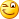## Sunday, September 4, 2011

### Completing the square

I have been thinking of posting some of my Mathematics and Physics lessons on this blog. These are lessons that, I have been using since 1997 during my private tutoring hours, improving over time, mostly for students studying for London General Certificate of Education, Ordinary Level [London GCE O’ Level] and Cambridge International General Certificate of Secondary Education, Ordinary Level [CIE IGCSE O’ Level]. As the very first Mathematics tutorial on this blog, today I am going to show how quadratic equations can be solved by employing completing the square method.

### A few assumptions

Before we begin, a few assumptions need to be made regarding the prerequisites for this tutorial as, it so happens that, without prior knowledge of elementary algebra, it will be a bit difficult to grasp ideas that will be conveyed in this lesson. Thus, I will simply assume that, the reader is familiar with and has basic knowledge and understanding of

1. Algebra
2. The concept of roots, more specifically square roots
3. Expansion of binomials and factoring of trinomials using the two identities:

$a + b 2 ≡ a 2 + 2 a b + b 2$

$a - b 2 ≡ a 2 - 2 a b + b 2$

4. Quadratic equations including their general form

### Completing the square

Before going in to solving equations, let us first see what completing the square is all about. For that, consider a simple trinomial and see how it can be changed to a perfect square by applying our knowledge of the first identity above. Consider this quadratic expression $x 2 + 4 x + 3$

Now, how can we turn this into a perfect square, just like the identity? By careful observation it can be seen that, the constant or the last term of a perfect square is always the square of one half the coefficient of the x term. So, it must have 4 as the constant term in this expression. But, it has a 3! How do we change the 3 into 4? Simple! By adding 1. But, by adding 1 to the expression, also changes it to a completely new one changing its meaning. To overcome that, we need to subtract one from it.

So that, the expression retains its original meaning and would now look like this: $x 2 + 4 x + 4 - 1$Now, ignoring –1, the first three terms of this expression is a perfect square trinomial, which can be expressed as a square of the binomial: $( x + 2 ) 2$

Therefore, the complete expression now looks like this: $( x + 2 ) 2 - 1$

This process of turning a quadratic expression into a complete square is, what really completing the square means.

### Solution of quadratic equations by completing the square

Let us consider the above example by equating it to zero, making it a quadratic equation.
$x 2 + 4 x + 3 = 0$
Going through the same steps as above we get
$( x + 2 ) 2 - 1 = 0$
Solving for $x$,
$x = ± 1 - 2$
Giving $x = - 1$  and   $x = - 3$

Note that, one can also get the same results by factoring using the difference of two squares identity after completing the square as, 1 is a square number.

### One more example using the second identity

Consider this quadratic equation: $x 2 - 6 x - 7 = 0$To complete the square we need to change the constant term to 9. To do that we add 16 to it and subtract 16 to keep the whole expression unchanged giving: $x 2 - 6 x + 9 - 1 6 = 0$

Taking -16 to RHS and completing square on LHS $( x - 3 ) 2 = 1 6$solve for x by taking roots on both sides $x - 3 = ± 1 6$

Therefore, $x = 7$  and   $x = - 1$

### Another example

In the two examples above coefficient of the first term is 1. Let us now see an example with a coefficient greater than 1.

Consider

$3 x 2 - 3 6 x - 3 9 = 0$

This time we will take the constant term to RHS and complete the square on LHS

$3 x 2 - 3 6 x = 3 9$

In order to make the coefficient of the first term one, divide the whole equation by 3

$x 2 - 1 2 x = 1 3$

Half the coefficient of middle term is -6 squaring it gives 36. This needs to be added to both sides of the equation.

$x 2 - 1 2 x + 3 6 = 1 3 + 3 6$

Simplifying

$x 2 - 1 2 x + 3 6 = 4 9$

Factoring LHS

$( x - 6 ) 2 = 4 9$

Solving for x gives, x = 13 and x = -1

That is all about it, for now. Good Luck!#### 1 comment:

1.